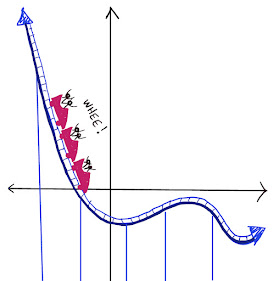## Thursday, March 25, 2021

### Toothpick Puzzle!

This is a repost of a problem from several years ago.  This time I am including an interactive document that you can use to move your toothpicks around and create your figures. First, make sure you are signed in with a Google/gmail account - otherwise you will be able to see the document but can't make your own copy.  Open the document and then under the "file" menu, click "make a copy" to make your own copy:  Toothpick Perimeter document

Here's the problem:

Notice that you can make figures with toothpicks.  We can think of each toothpick as a unit of length.  Below I made a figure with a perimeter of 12 and an area of 9:

Question:  How many different areas can be made with the same perimeter of 12?  (Notice that we can make a perimeter of 8 simply by "bending in" a corner of the figure. The area is smaller but the perimeter is the same.)  What is the smallest area you can make with 12 toothpicks?  The largest?  Can you make every whole number in between?

(This activity was inspired by a puzzle in Kordemsky's The Moscow Puzzles.)

For the solution, click "Read More" below.

Solution:

If we stick to rectilinear figures (figures whose sides meet at right angles), we can make areas as small as 5 and areas as large as 9, including every whole number in between.

Notice that a 3-4-5 triangle also has a perimeter of 12, and its area is 6.

Notice also that we can also use the strategy of starting with a small figure, such as the one in the bottom left of the figure below, which has an area of 4, and "bending in" one of the sides.  As long as we "bend out" the other side in exactly the same way, we haven't changed the area.  So that figure has an area of 4.  Using that strategy, you can create figures with areas even smaller than 4 with the same 12 toothpicks.